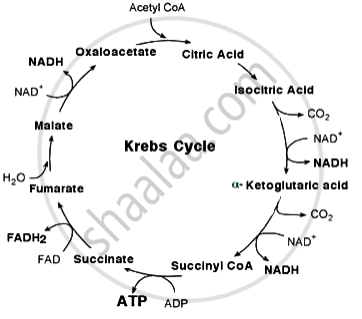# With the help ofschematic representation explain Krebs cycle. - Energy Relations - Number of ATP Molecules Generated

With the help ofschematic representation explain Krebs cycle.

#### Solution

Krebs cycle:-
The cyclic process through which acetyl Co-A is completely oxidised and CO2 is released in a step-wise manner is called Krebs cycle.

Steps in Krebs cycle:-

1.Condensation:

Acetyl coenzyme -A reacts with oxalo-acetate which is a 4-C compound present in matrix of mitochondrion
and a 6-C compound called citric acid or citrate is formed Thus citrate is the first stable compound of Krebs
cycle. One water molecules is used in this reaction. The enzyme citrate synthetase catalyses the reaction.

$\ce{\underset{\text{(4 - C)}}{OAA}+\underset{\text{(2 - C)}}{Acetyl Co-A} + H2O->[Citrate synthetase]\underset{\text{(6 - C)}}{Citrate} + Co - A}$

2. Isomerisation:

In the next step 6-C citrate is first converted into 6-C, Cis aconitate (removel of H2O) and then into 6-C

$\ce{\underset{\text{(6 - C)}}{Citrate}-> Cis - \underset{\text{(6 - C)}}{aconitate} + H2O}$

$\ce{Cis - \underset{\text{(6 - C)}}{aconitate} + H2O -> Iso \underset{\text{(6 - C)}}{citrate}}$

3.Oxidation :

Next reaction involves oxidation of Isocitrate (by removal of hydrogen) to form 6-C oxalo succinate. NADH2
is formed in this reaction.

$\ce{Iso - \underset{\text{(6 - C)}}{citrate} + NAD -> Oxalo - \underset{\text{(6 - C)}}{succinate} + NADH2}$

4. Decyroboxylation :-

Decarboxylation of 6-C oxalo succinate. Results in the formation of 5-C a-Ketoglutarate with the liberation
of CO2 .

$\ce{\underset{\text{(6 - C)}}{Oxalo-succinate}->\alpha - ketoglutarate + CO2}$

5. Oxidative Decyroboxylation :-

α - kelogutarate acid (5 - C) then undergoes oxidation (by removal of hydrogen) Decyroboxylation to
form 4 C succinyl Co - A : It takes place in the presence of Co - A and NAD. NADH2  is formed and CO2 is released.

$\ce{\underset{\text{(5C)}}{\alpha-ketoglutarate} + NAD + Co - A->\underset{\text{(4 - C)}}{SuccinylCo} - A + NADH2 + CO2 }$

6. Hydration and phosphorylation:

4-C succinyl-CoA is hydrolysed to succinate (4-C) in the next step. One H2O is used and Co-A is regenerated.
The reaction is exergonic. Energy released is used for the formation of GTP (Guanosine triphosphate) from
GDP and H3PO4 . Subsequently GTP is converted to ATP in presence of ADP.

$\ce{\underset{\text{(4 - C)}}{Succinyl Co} - A + H2O->[GDP -> GTP]\underset{\text{(4 - C)}}{Succinate} + Co - A}$

7. Oxidation (Dehydrogenation-III):

In this step, succinate (4-C) is oxidized by dehydrogenation to form fumarate (4-C). The hydrogen removed in this reaction reduces the coenzyme FAD (Flavin Adenine Dinucleotide) to FADH2 .The reaction is catalyzed by the enzyme succinate dehydrogenase.

$\ce{\underset{\text{(4 - C)}}{Succinate} + FAD->\underset{\text{(4 - C)}}{Fumarate} + FADH2}$

8. Hydration:

Fumarate (4-C) accepts a water molecule to produce malate (4-C). This occurs in presence of the enzyme
fumarase.

$\ce{\underset{\text{(4 - C)}}{Fumarate} + H2O ->\underset{\text{(4 - C)}}{Malate}}$

9. Oxidation (Dehydrogenation-IV):

Malate (4 - C) is oxidized by removal of hydrogen and oxalo-acetate (4 - C) gets regenerated. The hydrogen
removed in this reaction is taken up by the coenzyme NAD to form NADH2. . The reaction is catalyzed by
the enzyme malate dehydrogenase.

$\ce{\underset{\text{(4 - C)}}{Malate} + NAD ->\underset{\text{(4 - C)}}{Oxalo-acetate} + NADH2}$Concept: Energy Relations - Number of ATP Molecules Generated
Is there an error in this question or solution?
Share